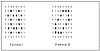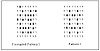# 66.C++ Neural Networks and Fuzzy Logic by Valluru B. Rao M&T Books, IDG Books Worldwide, Inc. ISBN: 1558515526   Pub Date: 06/01/95

The example of Yu and Mears, consists of eight 8-component vectors in both directions of the Bidirectional Associative Memory model. You can take these vectors, as they do, to define the pixel values in a 8x8 grid of pixels. What is being accomplished is the association of two spatial patterns. In terms of the binary numbers that show the pixel values, the patterns are shown in Figure 8.2. Call them Pattern I and Pattern II.Figure 8.2  Two patterns, Pattern I and Pattern II, given by pixel values: 1 for black, 0 for white.

Instead of Pattern I, they used a corrupted form of it as given in Figure 8.3. There was no problem in the network finding the associated pair (Pattern I, Pattern II).Figure 8.3  Pattern I and corrupted Pattern I.

In Figure 8.3, the corrupted version of Pattern I differs from Pattern I, in 10 of the 64 places, a corruption of 15.6%. This corruption percentage is cited as the limit below which, Yu and Mears state, heteroassociative recall is obtained. Thus noise elimination to a certain extent is possible with this model. As we have seen with the Hopfield memory, an application of associative memory is pattern completion, where you are presented a corrupted version of a pattern and you recall the true pattern. This is autoassociation. In the case of BAM, you have heteroassociative re call with a corrupted input.

### Summary

In this chapter, bidirectional associative memories are presented. The development of these memories is largely due to Kosko. They share with Adaptive Resonance Theory the feature of resonance between the two layers in the network. The bidirectional associative memories (BAM) network finds heteroassociation between binary patterns, and these are converted to bipolar values to determine the connection weight matrix. Even though there are connections in both directions between neurons in the two layers, essentially only one weight matrix is involved. You use the transpose of this weight matrix for the connections in the opposite direction. When one input at one end leads to some output at the other end, which in turn leads to output that is the same as the previous input, resonance is reached and an associated pair is found.

The continuous bidirectional associative memory extends the binary model to the continuous case. Adaptive bidirectional memories of different flavors are the result of incorporating different learning paradigms. A unipolar binary version of BAM is also presented as an application of the BAM for pattern completion.C++ Neural Networks and Fuzzy Logic
ISBN: 1558515526
EAN: 2147483647
Year: 1995
Pages: 139

Similar book on Amazon